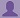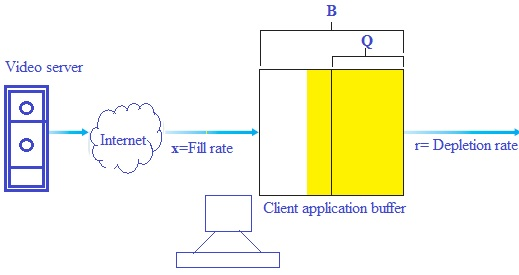SHARE
HELP

To start with, Sr2Jr’s first step is to reduce the expenses related to education. To achieve this goal Sr2Jr organized the textbook’s question and answers. Sr2Jr is community based and need your support to fill the question and answers. The question and answers posted will be available free of cost to all.# Computer Networking : A Top-down Approach

Authors:
James F. Kurose, Keith W. Ross
Exercise:
Problems
Chapter:
Multimedia Networking
Edition:
6
ISBN:
9780132856201
Question:
2
 Previous Next

###Question

Recall the simple model for HTTP streaming shown in Figure 7.3. Recall that B denotes the size of the client’s application buffer, and Q denotes the num- ber of bits that must be buffered before the client application begins playout. Also r denotes the video consumption rate. Assume that the server sends bits at a constant rate x whenever the client buffer is not full.

a. Suppose that x < r. As discussed in the text, in this case playout will alter- nate between periods of continuous playout and periods of freezing. Determine the length of each continuous playout and freezing period as a function of Q, r, and x.

b. Now suppose that x > r. At what time t = tf does the client application buffer become full?

###Answer

a)

Consider the analysis of client-side buffering for video streaming(figure 7.3):Now assume ‘B’ represents the size of the client’s application buffer, and ‘Q’ represents the number of bits and ‘r’ indicates the video consumption rate in above figure.

There must be buffered before the client application begins play out while begins with Q bits and rate decreases at ‘r-x’ times

Assume that the server sends bits at a constant rate ‘x’ and x < r, if the client buffer is not full.  The playout will alternate between periods of continuous playout and periods of freezing is seconds.

Therefore, the freezing period is  seconds.

b)

Assume that x > r.

If the client application buffer become full, then the freezing time period Q/X  to add additional B-Q bits.

That is

### Discussions

Post the discussion to improve the above solution.